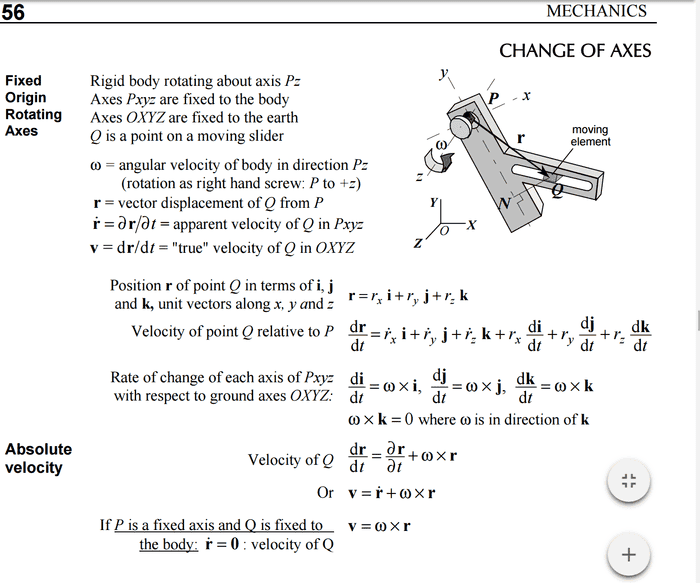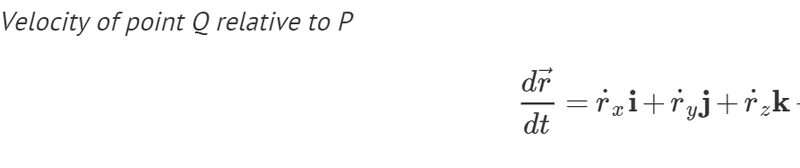The ''apparent'' velocity in an axis fixed to the bodyThe above states that the velocity of point Q relative to P is represented by dr/dt. However, it also states that ∂r/∂t is the apparent velocity of Q in Pxyz. How does that make sense? I mean P is the origin of axes Pxyz so surely the velocity of point Q relative to P should be the same as the apparent velocity of Q in Pxyz?

Also, slightly unrelated to the above, what is the exact difference between a frame of reference and an axis? My lecturer uses them interchangeably but I suspect there is a difference.

I'm a layman when it comes to this topic and my lecturer hasn't explained this whole topic very well so I'd appreciate it if someone could simplify.

Last edited:

BvU
Homework Helper
Oh boy, book's not so clear and teacher hasn't succeeded in lifting the fog.

True velocity of Q in OXYZ is the change of the vector OQ per delta time in the limit ##\Delta t \rightarrow 0##.
Now, vector OQ is equal to vector OP + ##\vec r## --- but vector ##\vec r## has to be expressed in OXYZ coordinates to actually find the coordinates in OXYZ. You see that done a few lines further down.

OP is constant in time (fixed axis), so ##{d\over dt}\, \vec {OP} = 0## and thereby $${d\over dt}\, \vec {OQ} = {d\over dt}\, \vec {OP} + {d\over dt}\, \vec r = {d \vec r \over dt}$$

I find the ##\displaystyle \dot{\vec r} ={\partial r \over \partial t}## an unfortunate way of expressing. ##\vec r## is a function of t only.

--

I find the ##\displaystyle \dot{\vec r} ={\partial r \over \partial t}## an unfortunate way of expressing. ##\vec r## is a function of t only.

--

Now you mention it, the notes accompanying the lecture slides did mention that the notation isn't strictly correct but is being used for convenience (or something to that effect).

Since apparent velocity = ∂r/∂t , and you're saying that r is a function of t only then doesn't that imply that strictly speaking apparent velocity also = dr/dt ?

Thanks

BvU
Homework Helper
Since apparent velocity = ∂r/∂t , and you're saying that r is a function of t only then doesn't that imply that strictly speaking apparent velocity also = dr/dt ?
Yes. Not just strictly, but any which way. Velocity is defined as ##\equiv {d\vec r\over dt}## in any frame of reference.

What they work out here is the velocity in two different frames of reference. And they make things worse by using "apparent" for things in frame Pxyz -- without quotes.

The fog is created created because they don't mention in which of the two frames of reference the expressions are worked out. For example :
Velocity of point Q relative to P $${d\vec r \over dt} =\dot r_x {\bf i} + \dot r_y {\bf j} + \dot r_z {\bf k} + r_x {d {\bf i} \over dt } + r_y {d {\bf j} \over dt } + r_z {d {\bf k} \over dt }$$
is in frame OXYZ ! (in frame Pxyx the ## {\bf i}, \ {\bf j}, \ {\bf k} \ ## are not moving!)

See also the last line in your picture: Q fixed to the body ##\Rightarrow \vec r ## is constant in frame Pxyz, so there the velocity is zero. ( ##\vec 0## ! )

The writers are so deep into the jargon that for them it is a problem to present the material in a didactically perfect manner. Victims are the newbies -- ironically it is those for whom the book is written.

So as a consolation: by the time you have built up some experience with this stuff, you won't notice the sloppiness any more-- until you become a helper for others.

Yes. Not just strictly, but any which way. Velocity is defined as ##\equiv {d\vec r\over dt}## in any frame of reference.

What they work out here is the velocity in two different frames of reference. And they make things worse by using "apparent" for things in frame Pxyz -- without quotes.

The fog is created created because they don't mention in which of the two frames of reference the expressions are worked out. For example :
is in frame OXYZ ! (in frame Pxyx the ## {\bf i}, \ {\bf j}, \ {\bf k} \ ## are not moving!)

See also the last line in your picture: Q fixed to the body ##\Rightarrow \vec r ## is constant in frame Pxyz, so there the velocity is zero. ( ##\vec 0## ! )

The writers are so deep into the jargon that for them it is a problem to present the material in a didactically perfect manner. Victims are the newbies -- ironically it is those for whom the book is written.

So as a consolation: by the time you have built up some experience with this stuff, you won't notice the sloppiness any more-- until you become a helper for others.

Ah that makes sense. So to summarise would it be correct to say that the velocity of Q relative to P in the frame Pxyz is(i.e. the exact same as in the frame OXYZ but without the di/dt, dj/dt and dk/dt terms)?

Thanks :)

BvU
•